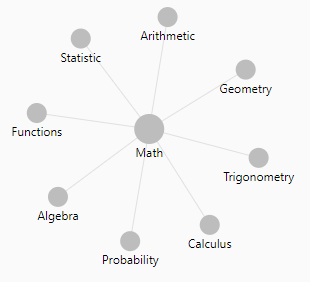# Mathematics - (Divisor|Denominator)

The divisor is an element of a division operation.

$\fracdividend}divisor} = quotient$

## Theorem

If d is a divisor of N then N/d is also a divisor of N.

## Type

### Trivial

If d is a divisor of N, d is trivial if its value is 1 or N.

## Greatest common

Euclid's algorithm for greatest common divisor. This algorithm that dates back some 2300 years is attributed to Euclid, and is very fast.

def gcd(x,y): return x if y == 0 else gcd(y, x % y)


Discover MoreInteger - Prime Number

A prime number is a positive integer greater than 1 that has no positive divisors other than 1 and itself. All prime numbers are , with one exception: the prime number 2. Python script that...Mathematics - (Prime Factorization Theorem | Factoring integers)

For every integer N >= 1, there is a unique bag of prime numbers whose product is N. All the elements in a bag must be prime. If N is itself prime, the bag for N is just {N}. 75 is the...Number - (Arithmetical | Numerical | Mathematical) Operators

Number operators Addition (+) Addition in an compound assignment form += Subtraction (-) Division (/) Python: Multiplication () Truncating integer division (//) ...Number - Division

A division is a mathematical binary function between 2 numbers. It is the definition of every rational number. where: The division symbol ÷ is just a blank fraction with dots replacing...
Number System - Rational Number -

A rational number is any number that can be expressed as the quotient or fraction p/q of two integers Rational numbers are also known as: the rationals, the field of rationals or the field of...